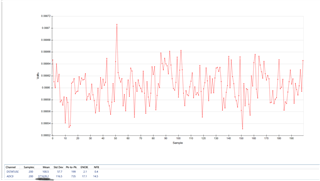If you have a related question, please click the "Ask a related question" button in the top right corner. The newly created question will be automatically linked to this question.I have my ADC0 mean value. I want to convert it into mean voltage value. What is the best way to do that. This is image for ADC evaluation software of time domain analysis.

• Hi Nikesh,

welcome to our e2e forum and thanks a lot for your interest in our ADS1220.

The EVM GUI should actually have a button where you can change the display from "codes" to "voltage".

If you want to convert codes to volts yourself then I usually recommend to calculate the LSB size (i.e. the voltage value represented by one code) first. You then multiply the LSB size with the code (given in decimal value) that you are reading.

LSB size = (2 x VREF) / (2^24 x Gain)

If you are e.g. using the internal VREF and a gain of 4 then the LSB size would be: (2 x 2.048V) / (2^24 x 4) = 61nV.
A code of 100 would then mean you have a applied a differential signal of 6.1uV at the inputs.

Regards,
Joachim Wuerker